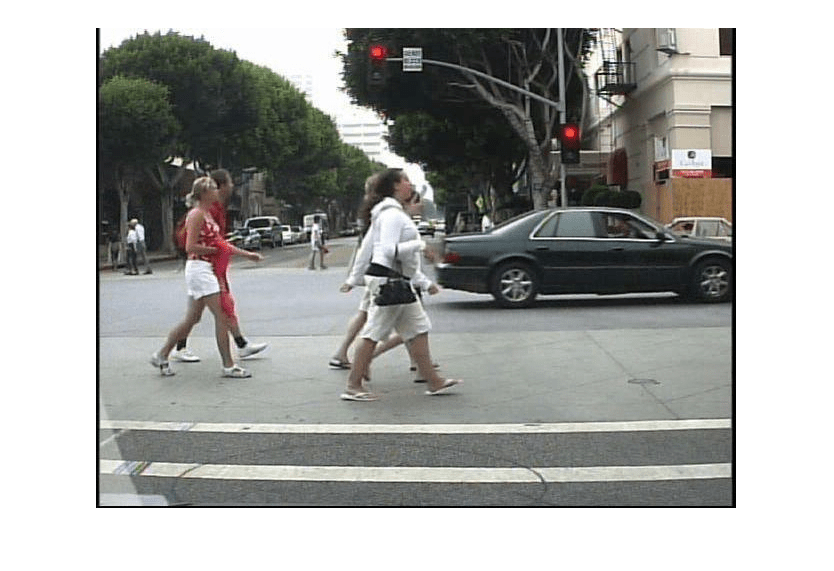Documentation

## Pedestrian Detection

This example shows code generation for pedestrian detection application that uses deep learning. Pedestrian detection is a key issue in computer vision. Pedestrian detection has several applications in the fields of autonomous driving, surveillance, robotics, and so on.

### Prerequisites

• CUDA® enabled NVIDIA® GPU with compute capability 3.2 or higher.

• NVIDIA CUDA toolkit and driver.

• NVIDIA cuDNN.

• Environment variables for the compilers and libraries. For information on the supported versions of the compilers and libraries, see Third-party Products (GPU Coder). For setting up the environment variables, see Setting Up the Prerequisite Products (GPU Coder).

• GPU Coder Interface for Deep Learning Libraries support package. To install this support package, use the Add-On Explorer.

### Verify GPU Environment

Use the `coder.checkGpuInstall` function to verify that the compilers and libraries necessary for running this example are set up correctly.

```envCfg = coder.gpuEnvConfig('host'); envCfg.DeepLibTarget = 'cudnn'; envCfg.DeepCodegen = 1; envCfg.Quiet = 1; coder.checkGpuInstall(envCfg); ```

### The Pedestrian Detection Network

The pedestrian detection network was trained by using images of pedestrians and non-pedestrians. This network is trained in MATLAB® by using the trainPedNet.m helper script. A sliding window approach crops patches from an image of size [64 32]. Patch dimensions are obtained from a heatmap, which represents the distribution of pedestrians in the images in the data set. It indicates the presence of pedestrians at various scales and locations in the images. In this example, patches of pedestrians close to the camera are cropped and processed. Non-Maximal Suppression (NMS) is applied on the obtained patches to merge them and detect complete pedestrians.The pedestrian detection network contains 12 layers which include convolution, fully connected, and classification output layers.

```load('PedNet.mat'); PedNet.Layers ```
```ans = 12x1 Layer array with layers: 1 'imageinput' Image Input 64x32x3 images with 'zerocenter' normalization 2 'conv_1' Convolution 20 5x5x3 convolutions with stride [1 1] and padding [0 0 0 0] 3 'relu_1' ReLU ReLU 4 'maxpool_1' Max Pooling 2x2 max pooling with stride [2 2] and padding [0 0 0 0] 5 'crossnorm' Cross Channel Normalization cross channel normalization with 5 channels per element 6 'conv_2' Convolution 20 5x5x20 convolutions with stride [1 1] and padding [0 0 0 0] 7 'relu_2' ReLU ReLU 8 'maxpool_2' Max Pooling 2x2 max pooling with stride [2 2] and padding [0 0 0 0] 9 'fc_1' Fully Connected 512 fully connected layer 10 'fc_2' Fully Connected 2 fully connected layer 11 'softmax' Softmax softmax 12 'classoutput' Classification Output crossentropyex with classes 'NonPed' and 'Ped' ```

### The `pedDetect_predict` Entry-Point Function

The pedDetect_predict.m entry-point function takes an image input and performs prediction on an image by using the deep learning network saved in the `PedNet.mat` file. The function loads the network object from the `PedNet.mat` file into a persistent variable pednet. Then function reuses the persistent object on subsequent calls.

```type('pedDetect_predict.m') ```
```function selectedBbox = pedDetect_predict(img) %#codegen % Copyright 2017-2019 The MathWorks, Inc. coder.gpu.kernelfun; persistent pednet; if isempty(pednet) pednet = coder.loadDeepLearningNetwork(coder.const('PedNet.mat'),'Pedestrian_Detection'); end [imgHt , imgWd , ~] = size(img); VrHt = [imgHt - 30 , imgHt]; % Two bands of vertical heights are considered % patchHt and patchWd are obtained from heat maps (heat map here refers to % pedestrians data represented in the form of a map with different % colors. Different colors indicate presence of pedestrians at various % scales). patchHt = 300; patchWd = patchHt/3; % PatchCount is used to estimate number of patches per image PatchCount = ((imgWd - patchWd)/20) + 2; maxPatchCount = PatchCount * 2; Itmp = zeros(64 , 32 , 3 , maxPatchCount); ltMin = zeros(maxPatchCount); lttop = zeros(maxPatchCount); idx = 1; % To count number of image patches obtained from sliding window cnt = 1; % To count number of patches predicted as pedestrians bbox = zeros(maxPatchCount , 4); value = zeros(maxPatchCount , 1); %% Region proposal for two bands for VrStride = 1 : 2 for HrStride = 1 : 20 : (imgWd - 60) % Obtain horizontal patches with stride 20. ltMin(idx) = HrStride + 1; rtMax = min(ltMin(idx) + patchWd , imgWd); lttop(idx) = (VrHt(VrStride) - patchHt); It = img(lttop(idx): VrHt(VrStride) , ltMin(idx) : rtMax , :); Itmp(:,:,:,idx) = imresize(It,[64,32]); idx = idx + 1; end end for j = 1 : size (Itmp,4) score = pednet.predict(Itmp(:,:,:,j)); % Classify ROI % accuracy of detected box should be greater than 0.90 if (score(1,2) > 0.80) bbox(cnt,:) = [ltMin(j),lttop(j), patchWd , patchHt]; value(cnt,:) = score(1,2); cnt = cnt + 1; end end %% NMS to merge similar boxes if ~isempty(bbox) [selectedBbox,~] = selectStrongestBbox(bbox(1:cnt-1,:),... value(1:cnt-1,:),'OverlapThreshold',0.002); end ```

### Generate CUDA MEX for the `pedDetect_predict` Function

Create a GPU Configuration object for a MEX target and set the target language to C++. Use the `coder.DeepLearningConfig` function to create a `CuDNN` deep learning configuration object and assign it to the `DeepLearningConfig` property of the GPU code configuration object. To generate CUDA MEX, use the `codegen` command and specify the size of the input image. This value corresponds to the input layer size of pedestrian detection network.

```% Load an input image. im = imread('test.jpg'); im = imresize(im,[480,640]); cfg = coder.gpuConfig('mex'); cfg.TargetLang = 'C++'; cfg.DeepLearningConfig = coder.DeepLearningConfig('cudnn'); codegen -config cfg pedDetect_predict -args {im} -report ```
```Code generation successful: To view the report, open('codegen/mex/pedDetect_predict/html/report.mldatx'). ```

### Run Generated MEX

Call `pednet_predict_mex` on the input image.

```imshow(im); ped_bboxes = pedDetect_predict_mex(im); ```Display the final predictions.

```outputImage = insertShape(im,'Rectangle',ped_bboxes,'LineWidth',3); imshow(outputImage); ```### Classification on Video

The included helper file pedDetect_predict.m grabs frames from a video, performs prediction, and displays the classification results on each of the captured video frames.

``` v = VideoReader('LiveData.avi'); fps = 0; while hasFrame(v) % Read frames from video im = readFrame(v); im = imresize(im,[480,640]);```
``` % Call MEX function for pednet prediction tic; ped_bboxes = pedDetect_predict_mex(im); newt = toc;```
``` % fps fps = .9*fps + .1*(1/newt);```
``` % display outputImage = insertShape(im,'Rectangle',ped_bboxes,'LineWidth',3); imshow(outputImage) pause(0.2) end```

Clear the static network object that was loaded in memory.

```clear mex; ```